Since visualization is still possible with one more dimension, suppose there are three links,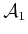,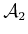, and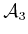. The C-space can be visualized as a 3D cube with opposite faces identified. Each coordinate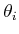ranges from 0 to, for which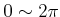. Suppose that each link has lengthto obtain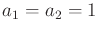. A point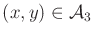is transformed as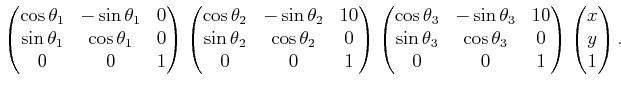(4.68)

To obtain polynomials, letand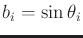, which results in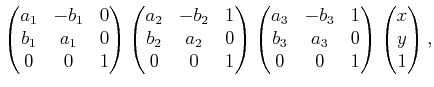(4.69)

for which the constraints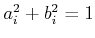for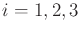must also be satisfied. This preserves the torus topology of, but now it is embedded in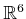. Multiplying the matrices yields the polynomials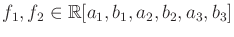, defined as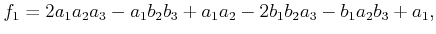(4.70)

and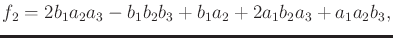(4.71)

for theand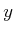coordinates, respectively.

Again, consider imposing a single constraint,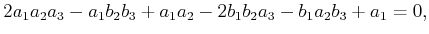(4.72)

which constrains the point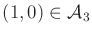to traverse the-axis. The resulting variety is an interesting manifold, depicted in Figure 4.24 (remember that the sides of the cube are identified).

Increasing the required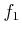value for the constraint on the final point causes the variety to shrink. Snapshots for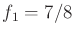and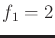are shown in Figure 4.25. At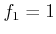, the variety is not a manifold, but it then changes to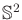. Eventually, this sphere is reduced to a point at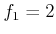, and then for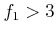the variety is empty.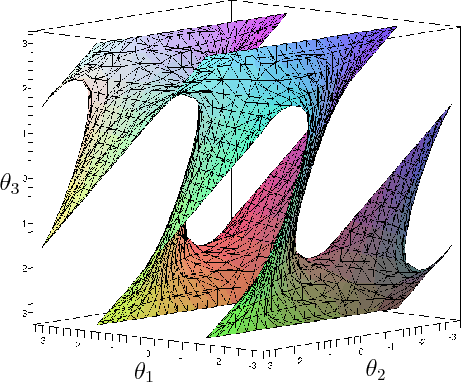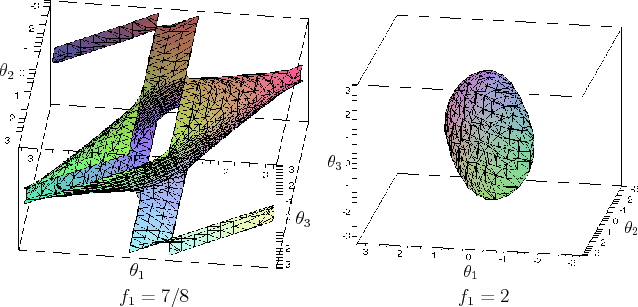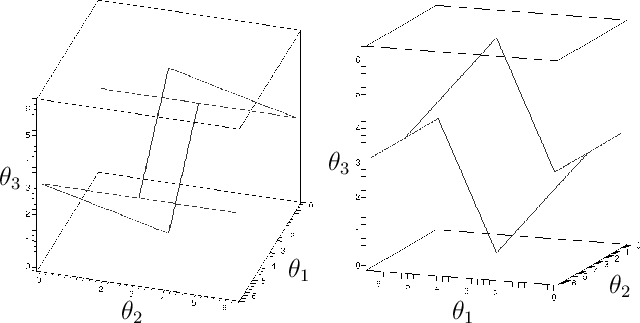Instead of the constraint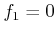, we could instead constrain thecoordinate of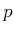to obtain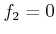. This yields another 2D variety. If both constraints are enforced simultaneously, then the result is the intersection of the two original varieties. For example, supposeand. This is equivalent to a kind of four-bar mechanism , in which the fourth link,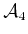, is fixed along the-axis from 0 to. The resulting variety,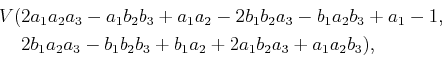(4.73)

is depicted in Figure 4.26. Using the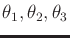coordinates, the solution may be easily parameterized as a collection of line segments. For all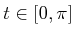, there exist solution points at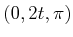,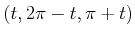,,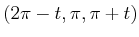, and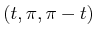. Note that once again the variety is not a manifold. A family of interesting varieties can be generated for the four-bar mechanism by selecting different lengths for the links. The topologies of these mechanisms have been determined for 2D and a 3D extension that uses spherical joints (see ).

Steven M LaValle 2020-08-14